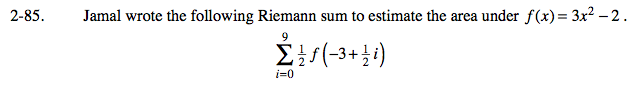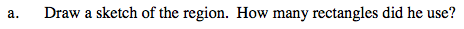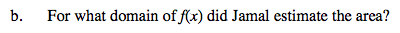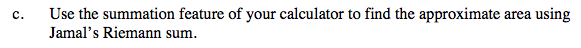### Home > CALC > Chapter Ch2 > Lesson 2.2.3 > Problem2-85

2-85.General form of left-endpoint Riemann Sum:

$\sum_{i=0}^{n-1}\Delta xf(a+\Delta xi)$

n − 1 = 9 n = 10 There are 10 rectangles.

The function is f(x) = 3x² − 2

a = −3 This is where the region starts.

$\Delta x=\frac{1}{2}$

$\text{Each rectangle has a width of }\frac{1}{2}.$Refer to the graph in part (a).Use the eTool below to complete the problem.
Click on the link to the right to view the full version of the eTool. Calc 2-85 HW eTool